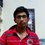# A problem in May Lunchtime 2014, Codechef.com

So i was trying to solve this problem.

 You are given a multiset of N integers. Please find such a nonempty subset of it that the sum of the subset's elements is divisible by N. Otherwise, state that this subset doesn't exist.


What i did was simply generate the combinations and check if each of them was divisible by its cardinality. This solution turned out just fine.

After the competetion i found an elegant solution here.

It so turns out that i cannot generate a sequence of n numbers without having a consecutive subset which is not a multiple of n.

That is to say, whatever combination of numbers i try..there always exists a consecutive subset which is a multiple of 'No. of numbers'(n).

Can anyone explain this theoritically? Or is there any theorem that states this?

PS: I already tried Googling it...all in vain.Note by Varshith Reddy
7 years, 2 months ago

This discussion board is a place to discuss our Daily Challenges and the math and science related to those challenges. Explanations are more than just a solution — they should explain the steps and thinking strategies that you used to obtain the solution. Comments should further the discussion of math and science.

When posting on Brilliant:

• Use the emojis to react to an explanation, whether you're congratulating a job well done , or just really confused .
• Ask specific questions about the challenge or the steps in somebody's explanation. Well-posed questions can add a lot to the discussion, but posting "I don't understand!" doesn't help anyone.
• Try to contribute something new to the discussion, whether it is an extension, generalization or other idea related to the challenge.

MarkdownAppears as
*italics* or _italics_ italics
**bold** or __bold__ bold
- bulleted- list
• bulleted
• list
1. numbered2. list
1. numbered
2. list
Note: you must add a full line of space before and after lists for them to show up correctly
paragraph 1paragraph 2

paragraph 1

paragraph 2

[example link](https://brilliant.org)example link
> This is a quote
This is a quote
    # I indented these lines
# 4 spaces, and now they show
# up as a code block.

print "hello world"
# I indented these lines
# 4 spaces, and now they show
# up as a code block.

print "hello world"
MathAppears as
Remember to wrap math in $$ ... $$ or $ ... $ to ensure proper formatting.
2 \times 3 $2 \times 3$
2^{34} $2^{34}$
a_{i-1} $a_{i-1}$
\frac{2}{3} $\frac{2}{3}$
\sqrt{2} $\sqrt{2}$
\sum_{i=1}^3 $\sum_{i=1}^3$
\sin \theta $\sin \theta$
\boxed{123} $\boxed{123}$

Sort by:

This is a classic application of Pigeonhole. Let the terms be $a_1$, $a_2$, $\dots$, $a_n$. Consider the $n$ sums \begin{aligned} s_1 &= a_1, \\ s_2 &= a_1 + a_2, \\ &\dots, \\ s_n &= a_1 + a_2 + \dots + a_n. \end{aligned} If any of these sums are divisible by $n$, then we are done.

Otherwise, each of these sums are congruent to one of 1, 2, $\dots$, $n - 1$ modulo $n$. There are $n$ sums and $n - 1$ possible residues modulo $n$, so by Pigeonhole, two residues must be the same. In other words, there exist $i$ and $j$, $i < j$, such that $s_i \equiv s_j \pmod{n}$. Then $s_j - s_i = a_{i + 1} + a_{i + 2} + \dots + a_j$ is divisible by $n$, as desired.

Furthermore, the example $a_1 = a_2 = \dots = a_{n - 1} = 1$ shows that you need at least $n$ terms to guarantee that you can find a sum of consecutive terms that are divisible by $n$.

- 7 years, 2 months ago

Oh. I first though to apply Pigeonhole to a different part of the problem. @Sreejato Bhattacharya remember that Pigeonhole trick/thing you showed me? By the way I'm totally showing that to the class. :D

- 7 years, 2 months ago

Cool! :)

- 7 years, 2 months ago

nice

- 7 years, 1 month ago

Interesting problem. Possibly a Pigeonhole argument is needed?

Staff - 7 years, 2 months ago

not a question

- 7 years, 1 month ago